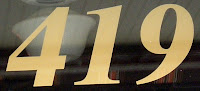## Monday, March 21, 2011

### 419

419 is a prime number.

419 is also a Sophie Germain prime because 2 x 419 + 1 = 839 is also prime. It is the 22nd Sophie Germain prime, and 22 = 2 x 11 is the product of two Sophie Germain primes.

419 and 421 form a twin prime pair.

419 is a divisor of 1311 - 1.

419 is the smallest number that when divided by 2, 3, 4, 5, 6, and 7 leaves remainders of 1, 2, 3, 4, 5, and 6, respectively. Also, 419 is 1 less than the least common multiple of the first seven integers.

419, 41, and 19 are all primes. Their reversals, 914, 14, and 91, are all semiprimes.Dutch naturalist and microscopy pioneer Anton van Leeuwenhoek (1632-1723) ground a total of 419 lenses during his life.